# Correlation property for sequences

Complex-valued-periodic sequences (i.e. sequences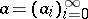with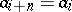,) with "good" correlation properties have many applications in signal processing (spread spectrum and code division multiple access communication systems, see [a10]). A good survey is [a9]. Periodic sequences are recurring or shift register sequences, see [a2].

Ifandare-periodic sequences, one defines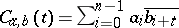. These numbers are called the periodic correlation coefficients (in case, periodic autocorrelation coefficients). Sometimes also the aperiodic correlation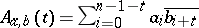is of interest. The odd correlation of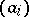and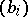is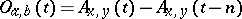,.

The goal is the design of large setsof-periodic sequences such that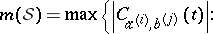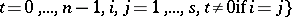(or the respective value for the aperiodic correlation) is small. Sequences with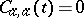,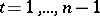, are called perfect. Perfect sequences whose entries areth roots of unity (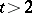) are known for many periods, but no example of a-sequence with period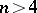is known (the perfect sequence conjecture: there are no perfect binary sequences with). In the aperiodic case, the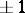-sequences with odd periodand aperiodic correlation coefficientsand(Barker sequences) have been classified [a12]. Ifis even, the existence of an-periodic Barker sequence implies the existence of an-periodic perfect-sequence.

If one assumes that the sequences are normalized, i.e.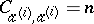, then it is known that for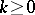,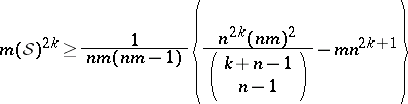and, if the entries of the sequences are just: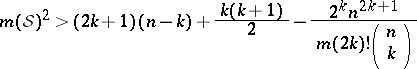for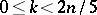. If the entries of the sequences areth roots of unity (), then the bound is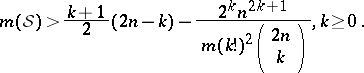The first bound is due to L.R. Welch, the second two bounds are due to V.M. Sidel'nikov. In all cases,is an integer. Some improvements of these bounds are known. Similar bounds hold for the maximum odd correlation coefficient [a7].

Important classes of sequences are derived from so-called-sequences, whereis a primitive element of the finite fieldand. The sequenceis defined over a finite field. The complex-valued sequences corresponding to such finite field sequences are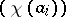, whereis a homomorphism from the additive group of the field into the multiplicative group of. The-sequences yield complex sequences with autocorrelation coefficients. The-decimation of an-periodic sequence is. Systematic investigations of the correlation properties between-sequences and their decimations can be found in [a4] and [a11]. The-sequences are recurring sequences of maximum period length. Their good autocorrelation properties show that they have good "random" properties (pseudo-noise sequences).

In many cases, variations of the construction of-sequences yield sets of sequences with optimal correlation properties with respect to the Welch and Sidel'nikov bounds: The cases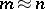and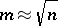have been investigated intensively. Regarding binary sequences, both the Gold sequences () and the Kasami sequences (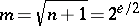) are asymptotically optimal. (One says that a family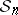of sets of-periodic sequences is asymptotically optimal if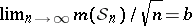, is best possible. If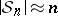, the lower bounds show that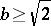for binary and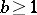for arbitrary complex valued sequences. For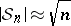, one has.) Sequences whose entries areth roots of unity () and which are optimal have been constructed (,prime [a5];,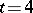[a1]). The latter construction is of particular interest in view of its connection with Kerdock and Preparata codes (cf. Kerdock and Preparata codes) and their linearity as codes over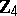, see [a3]. More sequences with good correlation properties whereis not a prime number can be constructed. In these constructions, sequences over Galois rings instead of finite fields are converted via homomorphismsinto complex-valued sequences. A systematic investigation of shift register sequences over rings can be found in [a6].

Other important classes of sequences with good correlation properties are bent function sequences, [a8].